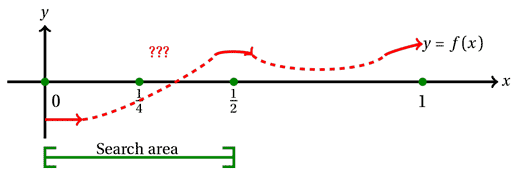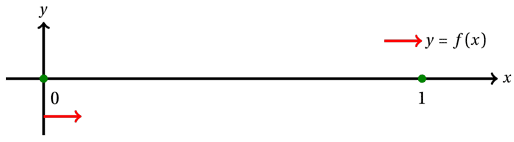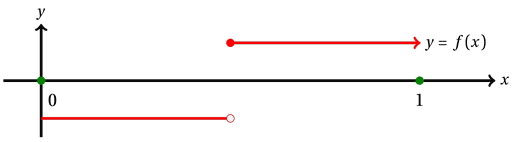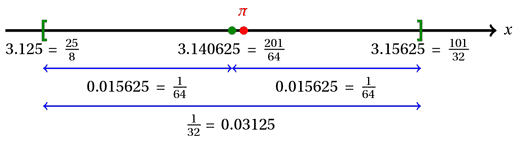## Content

### The bisection method

Be approximately right rather than exactly wrong.

— John W. Tukey

To "bisect" something means to cut it in half. The bisection method searches for a solution by bisecting: narrowing down the search area by half at each step.

The idea is as follows. Suppose you want to solve an equation $f(x)=0$, and you know there's a solution somewhere between $0$ and $1$. For instance, suppose you know that $f(0)$ is negative, while $f(1)$ is positive. Then there must be a solution to the equation somewhere between $0$ and $1$! Your search area is the interval $[0,1]$.

We've drawn the situation below. The graph of $y=f(x)$ is drawn in red, but the dotted part is unknown; all we know is that the graph is a curve connecting the parts drawn in solid red. The search area is marked in green.You examine the centre of the search area, evaluating $f(\frac{1}{2})$. If $f(\frac{1}{2}) = 0$, you have a solution, and you're done! Otherwise, $f(\frac{1}{2}) \neq 0$. In this case $f(\frac{1}{2})$ could be positive or negative.

First, suppose $f(\frac{1}{2})$ is positive. Then $f(x)$ must change sign between $f(0)$, which is negative, and $f(\frac{1}{2})$, which is positive. So there must be a solution between $0$ and $\frac{1}{2}$. (There could be other solutions as well, but we only need one!) You've narrowed down your search area to $[0,\frac{1}{2}]$, as shown.Alternatively, $f(\frac{1}{2})$ could be negative. In this case, $f(x)$ changes sign between $f(\frac{1}{2})$ (which is negative) and $f(1)$ (which is positive). So there must be a solution between $\frac{1}{2}$ and $1$. There could be other solutions as well, but there's certainly one between $\frac{1}{2}$ and $1$. You've narrowed down your search area to $[\frac{1}{2}, 1]$, as shown.Either way, your search area has been narrowed down from $[0,1]$, an interval of length $1$, to a smaller interval, of length $\frac{1}{2}$.

You now continue searching. You take $x$ to be in the middle of your new search area. If your search area is now $[0,\frac{1}{2}]$, you try $x=\frac{1}{4}$. If your search area is now $[\frac{1}{2}, 1]$, you try $x=\frac{3}{4}$. If you find $f=0$ at this point, you have a solution! If you don't, you narrow down your search area by half again.

Proceeding in this way, you'll either find a solution, or get very close to one. How close? As close as you like. However closely you want to approximate a solution, you'll be able to do it with the bisection algorithm.

For instance, suppose that your equation $f(x) = 0$ had a solution precisely at $x = \frac{1}{\pi} \sim 0.3183$. (You don't know that of course; you're trying to find the solution!) You'd first narrow down the interval from $[0,1]$ to $[0, \frac{1}{2}]$; then to $[\frac{1}{4}, \frac{1}{2}] = [0.25, 0.5]$; then to $[\frac{1}{4}, \frac{3}{8}] = [0.25, 0.375]$; then to $[\frac{5}{16}, \frac{3}{8}] = [0.3125, 0.375]$; and so on. See the figure below.Having described the idea of the bisection method, we'll next discuss the theory behind it more rigorously.

#### Intermediate value theorem

The bisection method relies upon an important theorem: the intermediate value theorem. This theorem is a very intuitive one. If you're on one side of a river, and later you're on the other side of the river, then you must have crossed the river!

Consider a function $f: [0,1] \rightarrow \mathbb{R}$ and its graph $y = f(x)$. If $f(0) < 0$, then the graph lies below the $x$-axis at $x=0$. If $f(1) > 0$, then the graph lies above the $x$-axis at $x=1$. (Portions of the graph must appear as shown below.) So between $x=0$ and $x=1$, the graph must cross the $x$-axis.But beware! If $f$ is discontinuous, the graph $y = f(x)$ could jump over the $x$-axis!Exercise 1

Find an example, with an explicit formula, of a function $f: [0,1] \rightarrow \mathbb{R}$ such that $f(0) < 0$, $f(1) > 0$, and for all $x \in [0,1]$, $f(x) \neq 0$.

Nonetheless, provided we stick with continuous functions, the graph must "cross the river" of the $x$-axis. That is, there must be an $x \in [0,1]$ such that $f(x) = 0$.

Now, although we described the left endpoint being below the river (i.e. $f(0) < 0$) and the right endpoint being above the river (i.e. $f(1) > 0$), it could be the other way around, and the same conclusion would hold. If $f(0)>0$ and $f(1)<0$, there still must be a solution $x \in [0,1]$ to $f(x)=0$.

There's nothing special about the interval $[0,1]$ either. We could replace $[0,1]$ with any interval $[a,b]$. Provided $f$ is positive at one end and negative at the other end, then $f(x)=0$ must have a solution in $[a,b]$.

Finally, there's also nothing special about the value $0$. For any real number value $c$, if $f < c$ at one end of the interval $[a,b]$, and $f>c$ at the other end, then there must exist an $x \in [a,b]$ such that $f(x) = c$.

We summarise this discussion with a statement of the intermediate value theorem.

###### Theorem (Intermediate Value Theorem)

Let $f : [a,b] \rightarrow \mathbb{R}$ be a continuous function, and $c$ be a real number.

1. If $f(a) < c$ and $f(b) > c$, then there exists an $x \in [a,b]$ such that $f(x) = c$.
2. If $f(a) > c$ and $f(b) < c$, then there exists an $x \in [a,b]$ such that $f(x) = c$.

Note that the intermediate value theorem doesn't say anything about how many times $f(x)$ takes the value $c$. There might be many values of $x$ in the interval $[a,b]$ such that $f(x) = c$. All the theorem says is that there is at least one.

If you're on one side of the river, and later you're on the other side of the river, then you must have crossed the river — you might have crossed it many times, but you certainly crossed it at least once!

#### Bisection algorithm

We now describe the bisection algorithm in detail. Suppose we have a continuous function $f: [a,b] \rightarrow \mathbb{R}$, and we want to solve $f(x) = 0$. (To solve for some other value of $f$, i.e. $f(x) = c$, you can rearrange the equation to $f(x) - c = 0$.)

We assume $f(a)$ and $f(b)$ are both nonzero — otherwise we already have a solution! We also assume $f(a)$ and $f(b)$ have opposite signs. The intermediate value theorem then ensures that $f(x) = 0$ has a solution for some $x \in [a,b]$.

As we have seen, the idea is to look at the value of $f(x)$ at the midpoint $\frac{a+b}{2}$ of the interval $[a,b]$, i.e. the average (or mean) of $a$ and $b$.

If $f( \frac{a+b}{2} ) = 0$, then we have a solution, and we can stop. Otherwise, $f( \frac{a+b}{2} )$ is either positive or negative. Depending on the signs of $f(a)$, $f(\frac{a+b}{2})$ and $f(b)$, the intermediate value theorem will tell us that one or the other of the intervals

$\left[ a, \frac{a+b}{2} \right] \quad \text{or} \quad \left[ \frac{a+b}{2}, b \right]$

contains a solution. We call this interval $[a_1, b_1]$.

We then repeat the process, evaluating $f$ at the midpoint $\frac{a_1 + b_1}{2}$ of $[a_1, b_1]$. If $f\left( \frac{a_1 + b_1}{2} \right) = 0$, we have a solution, and stop. Otherwise, $f \left( \frac{a_1 + b_1}{2} \right) \neq 0$ and the intermediate value theorem tells us that one or the other of the two sub-intervals obtained by bisecting $[a_1, b_1]$ contains a solution. We call this interval $[a_2, b_2]$. We continue in this fashion, obtaining intervals $[a_3, b_3]$, $[a_4, b_4]$, and so on.

We now state the bisection method rigorously.

#### Bisection method

Let $f: [a,b] \rightarrow \mathbb{R}$ be a continuous function with $f(a)$ and $f(b)$ both nonzero, and of opposite sign. We seek a solution of $f(x) = 0$.

Let $[a_0, b_0] = [a,b]$. At the $n$'th step of the method, we have an interval $[a_{n-1}, b_{n-1}]$, where $f(a_{n-1})$ and $f(b_{n-1})$ are both nonzero and of opposite signs. Then:

1. Calculate $f \left( \frac{ a_{n-1} + b_{n-1} }{2} \right)$. If $f \left( \frac{a_{n-1} + b_{n-1}}{2} \right) = 0$, $x = \frac{a_{n-1} + b_{n-1}}{2}$ is a solution, and we stop.
2. Otherwise, $f \left( \frac{a_{n-1} + b_{n-1}}{2} \right) \neq 0$. Precisely one of the following two possibilities occurs:
• $f \left( \frac{a_{n-1} + b_{n-1}}{2} \right)$ and $f(a_{n-1})$ have opposite signs. Then let $[a_{n}, b_{n}] = \left[ a_{n-1}, \frac{a_{n-1} + b_{n-1}}{2} \right]$.
• $f \left( \frac{a_{n-1} + b_{n-1}}{2} \right)$ and $f(b_{n-1})$ have opposite signs. Then let $[a_{n}, b_{n}] = \left[ \frac{a_{n-1} + b_{n-1}}{2} , b_{n-1} \right]$.
1. If the interval $[a_{n}, b_{n}]$ has the desired degree of accuracy for a solution, stop. Otherwise, return to step 1 and continue.
Note that the intervals $[a_n, b_n]$ produced by the bisection method are all nested: $[a_0,b_0] \supset \left[ a_1, b_1 \right] \supset \left[ a_2, b_2 \right] \supset \cdots.$

Moreover, each of these intervals is half as long as the previous one: $[a_n, b_n]$ is half the length of $[a_{n-1}, b_{n-1}]$.

Exercise 2

How many times smaller is the length of the interval $[a_n, b_n]$ compared to the original $[a,b] = [a_0, b_0]$? In other words, what is

$\frac{b_n - a_n}{b-a} ?$

#### Using the bisection algorithm

Let's now turn to some examples. We'll obtain some approximations to some well-known irrational numbers using the bisection method.

#### Example

Find $\pi$ to within an accuracy of $0.05$ by approximating the solution to $\sin x = 0$ in the interval $[3,4]$ using the bisection method.

Solution

Let $f(x) = \sin x$, so we apply the bisection method to $f$, starting from $[a_0, b_0] = [3,4]$. As $f$ is continuous, $f(3) = \sin 3 \sim 0.141$ is positive, and $f(4) = \sin 4 \sim -0.757$ is negative, so there is a solution in $[a_0,b_0]$.

We know from trigonometry that $\sin \pi = 0$, so $x = \pi$ is a solution. In fact, $\pi$ is the only solution for $x \in [3,4]$. So by approximately solving $\sin x = 0$ in $[3,4]$, we approximate $\pi$.

At the first step, we consider the midpoint $\frac{7}{2} = 3.5$ of $[a_0, b_0] = [3,4]$ and compute $f \left( \frac{7}{2} \right) = \sin 3.5 \sim -0.351$, which is negative. So the sign of $f(3)$, which is positive, is opposite to the sign of $f \left( \frac{7}{2} \right)$, which is negative. Hence by the intermediate value theorem, there must be a solution for $x \in [3, \frac{7}{2}]$, and we set $[a_1, b_1] = \left[ 3, \frac{7}{2}\right] = [3, 3.5]$.

At the second step, consider the midpoint $\frac{13}{4} = 3.25$ of $[a_1, b_1] = \left[ 3, 3.5 \right]$. We compute $f \left( \frac{13}{4} \right) = \sin 3.25 \sim -0.108$, which has opposite sign to $f(3)$, which is positive, and so we set $[a_2, b_2] = [3, \frac{13}{4}] = [3, 3.25]$.

At step 3, consider the midpoint $\frac{25}{8} = 3.125$ of $[a_2, b_2] = [3, 3.25]$ and compute $f \left( \frac{25}{8} \right) = \sin 3.125 \sim 0.017$, which has opposite sign to $f(3.25)$. So we take $[a_3, b_3] = \left[ \frac{25}{8}, \frac{13}{4} \right] = [3.125, 3.25]$.

At step 4, consider $\frac{51}{16} = 3.1875$. We compute $f(3.1875) = \sin 3.1875 \sim -0.046$, which has opposite sign to $f(3.125)$, so $[a_4, b_4] = \left[ \frac{25}{8}, \frac{51}{16} \right] = [3.125, 3.1875]$.

At step $5$, we compute $f \left( \frac{101}{32} \right) = f(3.15625) = \sin 3.15625 \sim -0.015$, which has opposite sign to $f(\frac{25}{8}) = f(3.125)$, so $[a_5, b_5] = \left[ \frac{25}{8}, \frac{101}{32} \right] = [3.125, 3.15625]$.

We now know that there is a solution to $\sin x = 0$ in the interval $\left[ \frac{25}{8}, \frac{101}{32} \right] = [3.125, 3.15625]$, which has length $\frac{101}{32} - \frac{25}{8} = \frac{1}{32} = 0.03125$. So we know the solution to within $0.03125$, which is better than the desired accuracy of $0.05$.

As you can see, the bisection method can be a rather repetitive and time-consuming process! Because it is an algorithm requiring repeated evaluation of a function, it is well suited to implementation on a computer.

We can save ourselves some effort by tabulating the computations. At each stage, we can note down $a_n$ and $b_n$ and the signs of $f(a_n)$ and $f(b_n)$, then the midpoint $\frac{a_n + b_n}{2}$ and the sign of $f( \frac{a_n + b_n}{2} )$. From this data we can calculate the next interval $[a_{n+1}, b_{n+1}]$ straightforwardly. This is quicker than writing out each step in English! The previous example could be tabulated as shown below.

Step $n$ $a_n$ Sign of $f(a_n)$ $b_n$ Sign of $f(b_n)$ Midpoint $\frac{a_n + b_n}{2}$ Sign of $f \left( \frac{a_n + b_n}{2} \right)$
$0$ $3$ $+$ $4$ $-$ $\frac{7}{2} = 3.5$ $-$
$1$ $3$ $+$ $\frac{7}{2} = 3.5$ $-$ $\frac{13}{4} = 3.25$ $-$
$2$ $3$ $+$ $\frac{13}{4} = 3.25$ $-$ $\frac{25}{8} = 3.125$ $+$
$3$ $\frac{25}{8} = 3.125$ $+$ $\frac{13}{4} = 3.25$ $-$ $\frac{51}{16} = 3.1875$ $-$
$4$ $\frac{25}{8} = 3.125$ $+$ $\frac{51}{16} = 3.1875$ $-$ $\frac{101}{32} = 3.15625$ $-$
$5$ $\frac{25}{8} = 3.125$ $+$ $\frac{101}{32} = 3.15625$ $-$

You might notice that in the column "Sign of $f(a_n)$", all the entries are the same, i.e. $+$; and in the column "Sign of $f(b_n)$", all the entries are also the same, i.e. $-$. In fact, the signs in these columns will never change.

Exercise 3

Prove that the signs of $f(a_0), f(a_1), f(a_2), \ldots, f(a_n)$ are all the same. Similarly, show that the signs of $f(b_0), f(b_1), f(b_2), \ldots, f(b_n)$ are all the same.

You might notice in our discussion that we continually write numbers both as fractions and decimals. Decimals are useful because they quickly convey how big each number is relative to the others. Fractions are useful because they express a number exactly and compactly.1 Of course you do not have to do the same, but it is useful to be aware of the advantages of writing numbers both ways.

1{Also, the author is a pure mathematician and cannot bear to see only decimals!}

#### Example

Find $\sqrt{2}$ to within an accuracy of $0.01$, by approximating the solution to $x^2 = 2$ in the interval $[1,2]$ using the bisection method.

Solution

Let $f(x) = x^2 - 2$, so we solve $f(x) = 0$ for $x \in [1,2]$ using the bisection method. The solutions to $x^2 - 2 = 0$ are $x = \pm \sqrt{2}$. Let $[a_0, b_0] = [1,2]$. As $f$ is continuous, $f(1)=-1<0$ and $f(2)=2>0$, there is a solution in this interval, i.e. $\sqrt{2}$. So we are approximating $\sqrt{2}$.

We tabulate the computations of the bisection method as follows.

 Step $n$ $a_n$ Sign of $f(a_n)$ $b_n$ Sign of $f(b_n)$ Midpoint $\frac{a_n + b_n}{2}$ Sign of $f \left( \frac{a_n + b_n}{2} \right)$ $0$ $1$ $-$ $2$ $+$ $\frac{3}{2} = 1.5$ $+$ $1$ $1$ $-$ $\frac{3}{2} = 1.5$ $+$ $\frac{5}{4}=1.25$ $-$ $2$ $\frac{5}{4}=1.25$ $-$ $\frac{3}{2} = 1.5$ $+$ $\frac{11}{8}=1.375$ $-$ $3$ $\frac{11}{8}=1.375$ $-$ $\frac{3}{2} = 1.5$ $+$ $\frac{23}{16}=1.4375$ $+$ $4$ $\frac{11}{8}=1.375$ $-$ $\frac{23}{16}=1.4375$ $+$ $\frac{45}{32}=1.40625$ $-$ $5$ $\frac{45}{32}=1.40625$ $-$ $\frac{23}{16}=1.4375$ $+$ $\frac{91}{64}=1.421875$ $+$ $6$ $\frac{45}{32}=1.40625$ $-$ $\frac{91}{64}=1.421875$ $+$ $\frac{181}{128}=1.4140625$ $-$ $7$ $\frac{181}{128}=1.4140625$ $-$ $\frac{91}{64}=1.421875$ $+$

After $7$ iterations, we know there is a solution to $x^2 - 2 = 0$ in the interval $[\frac{181}{128}, \frac{91}{64}]$, which has length $\frac{91}{64} - \frac{181}{128} = \frac{1}{128} = 0.0078125 < 0.01$. So we have determined the solution $\sqrt{2}$ to within an accuracy of $0.01$: it is between $1.4140625$ and $1.421875$.

These computations may appear quite exhausting! It took $7$ iterations to obtain $\sqrt{2}$ to within $0.01$, and we still do not know the second decimal digit of $\sqrt{2}$!

Exercise 4

Find $e$ to within an accuracy of $0.02$ by solving $\ln x = 1$ for $x$ in the interval $[2,3]$.

Exercise 5

How many solutions $x$ are there to the equation $\cos x = x$? Find all of them to within an accuracy of $0.01$, using the bisection method.

Exercise 6

Approximate $\frac{1}{3}$ to within an accuracy of $0.001$ by solving $3x-1 = 0$ for $x \in [0,1]$ using the bisection method. What do you note about the signs of $f \left( \frac{a_n + b_n}{2}\right)$?

(This last exercise might seem silly: you know how to express $1/3$ as a decimal! But an interesting pattern appears, which we discuss in the Links Forward section.)

#### How accurate is accurate?

In our first example, we found $\pi$ to an accuracy of $\frac{1}{32} = 0.03125$, after 5 iterations of the bisection method: $\pi$ is somewhere between 3.125 and 3.15625.

However, based on that example, what would be our best guess for $\pi$? We could guess the midpoint of the interval $[3.125, 3.15625]$, which is $3.140625$. How close is our best guess?

The situation is illustrated below.(We've illustrated the actual value of $\pi$ in red, but we're not supposed to know that!)

Well, if our best guess is too high, then as the solution can't be less than $\frac{25}{8} = 3.125$, the guess can't be off by more than $\frac{201}{64} - \frac{25}{8} = \frac{1}{64} = 0.015625$.

And if our best guess is too low, then as the solution can't be more than $\frac{101}{32} = 3.15625$, the guess can't be off by more than $\frac{101}{32} - \frac{201}{64} = \frac{1}{64} = 0.015625$.

Either way, we know that our best guess of $\frac{201}{64} = 3.140625$ is within $\frac{1}{64} = 0.015625$ of a solution. In this sense, the bisection method actually gave us an answer that was accurate to within $\frac{1}{64} = 0.015625$, twice as good as we originally suggested.

Exercise 7

Previously we approximated $\sqrt{2}$ by performing seven iterations of the bisection method. What is our best guess for $\sqrt{2}$? How close is it to the solution?

While we have been measuring accuracy of our solution by the length of $[a_n, b_n]$, often an approximation is required to be accurate to a number of decimal places.

To check that you have a solution to a desired number $k$ of decimal places, you should check that $a_n$ and $b_n$ agree to $k$ decimal places.

For example, our approximation of $\pi$ above does not even determine $\pi$ to one decimal place! Based on the interval $[3.125, 3.15625]$, to one decimal place, $\pi$ could be 3.1 or 3.2. To obtain $\pi$ to one decimal place, in fact, takes 7 iterations of the bisection method; but to obtain the second decimal place takes only one further iteration.

Exercise 8

Continue the example above computing an approximation to $\pi$, up to the eighth iteration of the bisection method, and verify that you can approximate $\pi$ to two decimal places.

For another example, when we approximated $\sqrt{2}$ above, it took $4$ iterations to find $\sqrt{2}$ to $1$ decimal place. It takes a further $7$ iterations to find $\sqrt{2}$ to $2$ decimal places!

Exercise 9

Continue the example above approximating to $\sqrt{2}$, up to $[a_{11}, b_{11}]$. Verify that it takes 11 iterations to approximate $\sqrt{2}$ to two decimal places.

Although the bisection method doubles its accuracy at each stage, its accuracy in terms of decimal places is much more haphazard!

We'll see later, in the Links Forward section, that there is a nice way to predict, based on the desired accuracy of our solution, just how many iterations of the bisection algorithm we need to calculate. On the other hand, we'll also see that, if we want a desired number of decimal places of accuracy, then it's harder to predict how many iterations of the bisection algorithm we will need.

#### Convergence of the bisection method

The best thing about the bisection method is that it is guaranteed to work. Provided you're using the method appropriately, you have a failsafe guarantee that the method works. That guarantee is the best possible type of guarantee: a mathematical theorem.

That is, if you're trying to solve $f(x) = 0$ in $[a,b]$, for a continuous function $f$, where $f(a)$ and $f(b)$ have opposite signs, then the bisection method is guaranteed to give you an arbitrarily good approximation to a solution.

"Arbitrarily good" means "as good as you want". You can say how good you want your approximation to be, and then, by applying the bisection method enough times, you can get the approximation you want.

More formally, suppose you say that you want your approximation to be accurate to within a number $\epsilon$ (the Greek letter epsilon). That is, you want to arrive at an interval $[a_n, b_n]$ where $b_n - a_n < \epsilon$. Then, by applying the bisection method enough times, you can obtain such an interval $[a_n, b_n]$ with $b_n - a_n < \epsilon$.

We can summarise the above formally as a theorem.

###### Theorem

Let $f: [a,b] \rightarrow \mathbb{R}$ be a continuous function and suppose $f(a)$, $f(b)$ are both nonzero and have opposite signs. Let $\epsilon$ be a positive number. Then the bisection method, after a finite number $N$ of iterations, will produce an interval $[a_N, b_N]$ such that $b_N - a_N < \epsilon$.

That is, after some finite number $N$ of iterations, we have a solution to $f(x)=0$ to within an accuracy of $\epsilon$.

Why is theorem true? The idea is simply that the lengths of the intervals $[a_n, b_n]$ halve at each stage. When you take a number and continually halve it, the number gets very small: as small as you like, smaller than any $\epsilon$ you prefer!

We'll see more details in the Links Forward section. There we will also see that there is a formula for $N$, the number of iterations of the bisection method required to obtain the desired accuracy.

The best thing about the bisection method may be that it is guaranteed to work, but the worst thing about the bisection method is that it is slow. We'll now see a method that is often much quicker in finding a solution — but not guaranteed to work every time.

Next page - Content - Newton's method